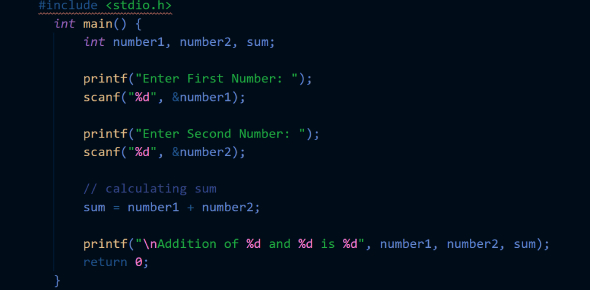# C Language Exam: Practice Quiz! Trivia

50 Questions | Attempts: 1679
ShareSettings.

• 1.
How would you copy the name “Hello” to a character array (i.e. string) declared as char str;
• A.

Strcpy( str, “Hello” );

• B.

Strcat(str,“Hello”);

• C.

Printf( str, “Hello” );

• D.

Str = “Hello”;

• 2.
count=0; for ( I=0;I<=10; I++) {if(I%2==0) count++; }printf(“%d”, count); Pick out the correct value for count
• A.

6

• B.

3

• C.

4

• D.

5

• 3.
The value within the [] brackets in an array declaration specifies
• A.

Value of the array element

• B.

Subscript value

• C.

• D.

Size of an array

• 4.
Compute the result of the following expression in ‘C’. A=3*4/5+10/5+8-1+7/8
• A.

11

• B.

10

• C.

12

• D.

9

• 5.
Dynamic memory allocation in array results in
• A.

Allocation of memory at debugging time

• B.

Allocation of memory at file saving time

• C.

Allocation of memory at compile time

• D.

Allocation of memory at runtime

• 6.
What is the output of the following module sum=0; I=0; do{ sum+=I; I++; }while(I<=5); printf(“%d”, sum);
• A.

28

• B.

10

• C.

15

• D.

21

• 7.
Which of the follwing is a string
• A.

‘abcd’

• B.

“abcd”

• C.

Abcd

• D.

‘abcd”

• 8.
Give the output of the following program: #include < stdio.h > main() {int I=1; while (I < 5) {printf(“%d”, I); }} /* End of Main */
• A.

Print the value of I as 1

• B.

Warning for no return type for main ( )

• C.

Infinite loop

• D.

Prints the value of I as11111

• 9.
What will happen if you try to put so many values into an array during the initalization such that its size is  exceeded
• A.

Error message from the compiler

• B.

Possible system malfunction

• C.

Last element data may be overwritten

• D.

Nothing

• 10.
Which of the following statements would read a single character from the keyboard and place the result in a character variable ‘ch’ defined as: char ch;
• A.

Ch = getch( );

• B.

Printf( “%c”, ch );

• C.

While(!kbhit)

• D.

Getkeyb ( ch );

• 11.
consider the array definition int num  = { 3 ,3 ,3 }; pick the correct answers
• A.

This is syntactically wrong

• B.

The value of num is 3

• C.

This is invalid if it comes within a function

• D.

The value of num is 3

• 12.
Output of the below program #include<stdio.h> main() {int a,b=0; int c={1,2,3,4,5,6,7,8,9,0 }; for(a=0;a<10;++a) b+=c[a]; printf(“%d”,b); }
• A.

1 3 6 10 15 21 28 32 45 45

• B.

55

• C.

45

• D.

0

• 13.
The maximum number of dimension an array can have in C language is
• A.

4

• B.

5

• C.

Compiler dependent

• D.

3

• 14.
What is the result of the expression ( 10/3 )*3+5%3 ?
• A.

10

• B.

11

• C.

8

• D.

1

• 15.
Array subscripts in ‘C’ always start at
• A.

0

• B.

Compiler dependent

• C.

1

• D.

-1

• 16.
The statements that prints out the character set from A-Z is, where a is an integer variable
• A.

For(a=‘A’; a

• B.

For(a=‘A’; a

• C.

For(a=‘a’; a

• D.

For(a=’A’ a

• 17.
The amount of storage required for holding elements of the array depends on
• A.

Data type

• B.

Datatype and size

• C.

Run-time requirement

• D.

Size

• 18.
If we don’t initialize a static array, what will be the elements set to:
• A.

Character constant

• B.

A floating point number

• C.

0

• D.

An undetermined value

• 19.
What is wrong with the following program main() { char m1= “message1”; char m2=“message2”; m2=m1; printf(“msg is %s”,m2); }
• A.

Char array cannot be printed directly using printf

• B.

Array is not a left value and so cannot be assigned to

• C.

Program compiles without error, but prints an unpredictable value

• D.

Array cannot be initialized as above

• 20.
The total memory required for an array
• A.

Sizeof (datatype) * 2

• B.

Size of (datatype) * size of used array elements

• C.

Sizeof (datatype) * sizeof array

• D.

Size of (array) * datatype

• 21.
How many bytes will be allotted for the declaration int num 
• A.

6 bytes

• B.

24 bytes

• C.

12 bytes

• D.

48 bytes

• 22.
If statement is a —————statement
• A.

Loop construct

• B.

Two way decision

• C.

Multiway decision

• D.

One-way decision

• 23.
What is the output of the following program main() {static int y; printf (“%d\n”, y); }
• A.

0

• B.

Run-time error

• C.

Undefined

• D.

Compilation error

• 24.
printf (“\ “ well done\” ”); what will be the output of this statement
• A.

\“well done \”

• B.

Well done

• C.

“ well done”

• D.

\ well done \

• 25.
Which of the following is not correct
• A.

While loop is executed atleast once

• B.

Do . while loop is executed at least once

• C.

While loop is executed only if the condition is true

• D.

Dowhile loop is ececuted only if the condition is true

## Related TopicsBack to top
×

Wait!
Here's an interesting quiz for you.